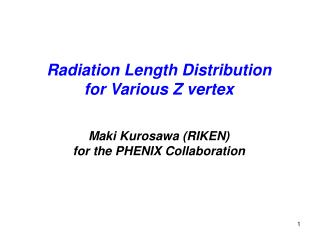DownloadDownload PresentationRadiation Length Distribution for Various Z vertex Maki Kurosawa (RIKEN)

# Radiation Length Distribution for Various Z vertex Maki Kurosawa (RIKEN)

Télécharger la présentation## Radiation Length Distribution for Various Z vertex Maki Kurosawa (RIKEN)

- - - - - - - - - - - - - - - - - - - - - - - - - - - E N D - - - - - - - - - - - - - - - - - - - - - - - - - - -
##### Presentation Transcript

1. Radiation Length Distribution for Various Z vertex Maki Kurosawa (RIKEN) for the PHENIX Collaboration

2. Radiation Length Tool Radiation length tool was worked (Hubert’s work). Details are at http://p25ext.lanl.gov/people/hubert/phenix/silicon/simulations/may08/lscan.html Create root file (PISA). http://p25ext.lanl.gov/people/hubert/phenix/silicon/simulations/jan07/geom_geant_root.html Need to type below root command to make radiation length plot. PHENIX->GetVolume(“SICG”)->LegoPlot(20,0,180, 60,0,360, 0,100) theta phi r

3. Radiation Length (with beam pipe) Beam Pipe Material : Beryllium Thickness : 500 mm Radiation Length : 35.28 cm radiation length v.s. phi v.s. theta radiation length v.s theta z = 0 cm 124 < phi (degree) < 214 radl Good match theta (degree) phi (degree) Using pass length Using radiation length tool

4. Radiation Length and PT Plots (w/o beam pipe) Applying cut of central arm acceptance. -0.35 < h < 0.35 -34 < Phi (degree) < 56 || 124 < Phi (degree) < 214 -15 < Vertex_z (cm) < 0 Radiation Length : 9.5% PT (GeV/c) PT (GeV/c) PT (GeV/c) PT (GeV/c)

5. Radiation Length and PT Plots (w/o beam pipe) Applying cut of central arm acceptance. -0.35 < h < 0.35 -34 < Phi (degree) < 56 || 124 < Phi (degree) < 214 +5 < Vertex_z (cm) < +15 PT (GeV/c) PT (GeV/c) PT (GeV/c)

6. Radiation Length and PT Plots (w/o beam pipe) NOT applying acceptance cut. 0 < theta (degree) < 180 0 < phi (degree) < 360 -15 < Vertex_z (cm) < 0 PT (GeV/c) PT (GeV/c) PT (GeV/c) PT (GeV/c)

7. Radiation Length and PT Plots (w/o beam pipe) NOT applying acceptance cut. 0 < theta (degree) < 180 0 < phi (degree) < 360 +5 < Vertex_z (cm) < +15 PT (GeV/c) PT (GeV/c) PT (GeV/c)

8. Back Up

9. Radiation Length Tool in gffgo.dat, change line SWIT 5 0 1 0 0 0 0 0 0 ! ... to SWIT 2 0 1 0 0 0 0 0 0 ! pisa g2root geometry.dat geometry.C root geometry.C root  gGeoManager->Export("geom.root", "PHENIX") create geom.root TH2F* h2; TProfile* pfx; TH1D* pfx_px; TFile* tf = new TFile( “geom.root” ); h2 = PHENIX->GetVolume(“SICG”)->LegoPlot(55., 65., 120., 90., 124., 214., 0., 20.); h2 = new TH2F(“h2”, “”, 80, 0, 180, 11, 4, 15); for( int theta = 1; theta < 56; theta++ ){ for( int phi = 1; phi < 91; phi++ ){ float rads = h2->GetBinContent(phi, theta)*100; h2->Fill( theta+64, rads ); } } pfx = h2->ProfileX(); pfx_px = pfx->ProjectionX(); pfx_px->Draw();

10. Radiation Length (without beam pipe) From my previous slide at VTX simulation meeting (31th Oct 2008) Beam pipe was not taken in account for calculation of radiation length. Support ring http://p25ext.lanl.gov/people/hubert/phenix/silicon/simulations/may08/lscan.html

11. Radiation Length (with beam pipe) Beam Pipe Material : Beryllium Thickness : 500 mm Radiation Length : 35.28 cm 124 < phi < 214 z = 0 cm http://p25ext.lanl.gov/people/hubert/phenix/silicon/simulations/may08/lscan.html

12. Calculation of Radiation Length AB BC Use of a pass length through medium to obtain a radiation length. Not use LSCAN or HSCAN A (x1, y1, z1) medium1 : radl = X0(1) B (x2, y2, z2) medium2 : radl = X0(2) C (x3, y3, z3) Radiation Length : Coordinates of point A, B, C... X0(1), X0(2)… can be obtained from PISA.